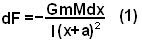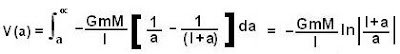## Monday, September 1, 2008

### Irodov Problem 1.206

Gravitational potential of a mass at some position in a gravitational field is the work done in moving the mass to infinity from that position. In short if the gravitational force is the vector F(r), r being the position vector then the gravitational potential at r is given by the integral

a) For two point particles of masses m1 and m2 it is then,b)Let us consider an infinitesimally small part of the rod of length dx. Its mass will be Mdx/l. The gravitational force of attraction between this infinitesimally small part of the rod and the point mass m will be,The total gravitational force acting between the rod and the point mass can be determined by integrating (1) over the entire rod given by,